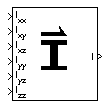# Symmetric Inertia Tensor

Create inertia tensor from moments and products of inertia

•Libraries:
Aerospace Blockset / Mass Properties

## Description

The Symmetric Inertia Tensor block creates an inertia tensor from moments and products of inertia. Each input corresponds to an element of the tensor.

The inertia tensor has the form of:

`$Inertia=\left[\begin{array}{lll}{I}_{xx}\hfill & -{I}_{xy}\hfill & -{I}_{xz}\hfill \\ -{I}_{xy}\hfill & {I}_{yy}\hfill & -{I}_{yz}\hfill \\ -{I}_{xz}\hfill & -{I}_{yz}\hfill & {I}_{zz}\hfill \end{array}\right]$`

## Ports

### Input

expand all

Moment of inertia about the x-axis, specified as a scalar.

Data Types: `double`

Product of inertia in the xy plane, specified as a scalar.

Data Types: `double`

Product of inertia in the xz plane, specified as a scalar.

Data Types: `double`

Moment of inertia about the y-axis, specified as a scalar.

Data Types: `double`

Product of inertia in the yz plane, specified as a scalar.

Data Types: `double`

Moment of inertia about the z-axis, specified as a scalar.

Data Types: `double`

### Output

expand all

Symmetric inertia tensor, returned as a 3-by-3 matrix.

Data Types: `double`

## Version History

Introduced before R2006a